Share

# Selina solutions for Concise Physics Class 9 ICSE chapter 2 - Motion in One Dimension [Latest edition]

Course
Textbook page

#### Chapters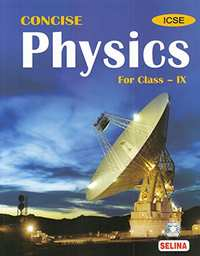## Chapter 2: Motion in One Dimension

OthersExercise - 2(A)Exercise - 2(B)Exercise - 2(C)

### Selina solutions for Concise Physics Class 9 ICSE Chapter 2 Motion in One Dimension Exercise - 2(A) [Page 35]

Exercise - 2(A) | Q 1 | Page 35

Differentiate between the scalar and vector quantities, giving two examples of each.

Exercise - 2(A) | Q 2 | Page 35

State whether the following quantity is a scalar or vector?

 a) pressure d) force b) momentum e) energy c) weight f) speed
Exercise - 2(A) | Q 3 | Page 35

When is a body said to be at rest?

Exercise - 2(A) | Q 4 | Page 35

When is a body said to be in motion?

Exercise - 2(A) | Q 5 | Page 35

What do you mean by motion in one direction?

Exercise - 2(A) | Q 6 | Page 35

Define displacement. State its unit.

Exercise - 2(A) | Q 7 | Page 35

Differentiate between distance and displacement.

Exercise - 2(A) | Q 8 | Page 35

Can displacement be zero even if the distance is not zero? Give one example to explain your answer.

Exercise - 2(A) | Q 9 | Page 35

When is the magnitude of displacement equal to the distance?

Exercise - 2(A) | Q 10 | Page 35

Define velocity. State its unit.

Exercise - 2(A) | Q 11 | Page 35

Define speed. What is its S.I. unit?

Exercise - 2(A) | Q 12 | Page 35

Distinguish between speed and velocity.

Exercise - 2(A) | Q 13 | Page 35

Which of the quantity-speed or velocity-gives the direction of motion of a body?

Exercise - 2(A) | Q 14 | Page 35

When is instantaneous speed the same as the average speed?

Exercise - 2(A) | Q 15 | Page 35

Distinguish between uniform velocity and variable velocity.

Exercise - 2(A) | Q 16 | Page 35

Distinguish between average speed and average velocity.

Exercise - 2(A) | Q 17 | Page 35

Give an example of the motion of a body moving with a constant speed but with a variable velocity. Draw a diagram to represent such a motion.

Exercise - 2(A) | Q 18 | Page 35

Give an example of motion in which the average speed is not zero but the average velocity is zero.

Exercise - 2(A) | Q 19 | Page 35

Define acceleration. State its unit.

Exercise - 2(A) | Q 20 | Page 35

Distinguish between acceleration and retardation.

Exercise - 2(A) | Q 21 | Page 35

Differentiate between uniform acceleration and variable acceleration.

Exercise - 2(A) | Q 22 | Page 35

What is meant by the term retardation? Name its S.I. unit.

Exercise - 2(A) | Q 23 | Page 35

Which of the quantity-velocity or acceleration-determines the direction of motion?

Exercise - 2(A) | Q 24.1 | Page 35

Give one example of following motion :

Uniform velocity

Exercise - 2(A) | Q 24.2 | Page 35

Give one example of following motion :

Variable velocity

Exercise - 2(A) | Q 24.3 | Page 35

Give one example of following motion :

Variable acceleration

Exercise - 2(A) | Q 24.4 | Page 35

Give one example of following motion :

Uniform retardation

Exercise - 2(A) | Q 25 | Page 35

The diagram below shows the pattern of the oil on the road at a constant rate from a moving car. What information do you get from it about the motion of the car.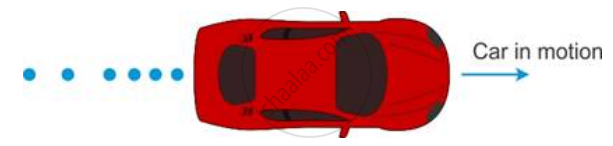Exercise - 2(A) | Q 26 | Page 35

Define the term acceleration due to gravity. State its average value.

Exercise - 2(A) | Q 27 | Page 35

'The value of g remains the same at all places on the Earth's surface'. Is this statement true? Give reason for your answer.

Exercise - 2(A) | Q 28 | Page 35

If a stone and a pencil are dropped simultaneously in vacuum from the top of a tower, then which of the two will reach the ground first? Give reason.

### Selina solutions for Concise Physics Class 9 ICSE Chapter 2 Motion in One Dimension Exercise - 2(A) [Pages 35 - 36]

Exercise - 2(A) | Q 1 | Page 35

The vector quantity is :

• Work

• Pressure

• Distance

• velocity

Exercise - 2(A) | Q 2 | Page 36

The S.I. unit of velocity is ___________

• km h-1

• m min-1

• km rnin-1

• m s-1

Exercise - 2(A) | Q 3 | Page 36

The unit of retardation is ____________

• m s-1

• m s-2

• m

• m s2

Exercise - 2(A) | Q 4 | Page 36

A body when projected up with an initial velocity u goes to a maximum height h in time t and then comes back at the point of projection. The correct statement is ______________

•  The average velocity is 2 h/t.

• The acceleration is zero.

• The final velocity on reaching the point of projection is 2 u.

• The displacement is zero.

Exercise - 2(A) | Q 5 | Page 36

18 km h-1 is equal to _____________

• 10 m s-1

• 5 m s-1

• 18 m s-1

• 1.8 m s-1

### Selina solutions for Concise Physics Class 9 ICSE Chapter 2 Motion in One Dimension Exercise - 2(A) [Page 36]

Exercise - 2(A) | Q 1 | Page 36

The speed of a car is 72 km h-1. Express it in m s-1.

Exercise - 2(A) | Q 2 | Page 36

Express 15 m s-1 in km h-1.

Exercise - 2(A) | Q 3.1 | Page 36

Express the following in m s-1.

1 km h-1

Exercise - 2(A) | Q 3.2 | Page 36

Express the following in m s-1.

18 km min-1

Exercise - 2(A) | Q 4 | Page 36

Arrange the following speeds in increasing order.

10 m s-1, 1 km min-1 and 18 km h-1.

Exercise - 2(A) | Q 5 | Page 36

A train takes 3 h to travel from Agra to Delhi with a uniform speed of 65 km h-1. Find the distance between the two cities.

Exercise - 2(A) | Q 6 | Page 36

A car travels the first 30 km with a uniform speed of 60 km h-1 and the next 30 km with a uniform speed of 40 km h-1. Calculate :
The total time of journey,
The average speed of the car.

Exercise - 2(A) | Q 7 | Page 36

A train takes 2 h to reach station B from station A, and then 3 h to return from station B to station A. The distance between the two stations is 200 km. Find:
The average speed,
The average velocity of the train.

Exercise - 2(A) | Q 8.1 | Page 36

A car moving on a straight path covers a distance of 1 km due east in 100 s. What is the speed of the car?

Exercise - 2(A) | Q 8.2 | Page 36

A car moving on a straight path covers a distance of 1 km due east in 100 s. What is velocity of the car?

Exercise - 2(A) | Q 9 | Page 36

A body starts from rest and acquires a velocity 10 m s-1 in 2 s. Find the acceleration.

Exercise - 2(A) | Q 10 | Page 36

A car starting from rest acquires a velocity 180m s-1 in 0.05 h. Find the acceleration.

Exercise - 2(A) | Q 11 | Page 36

A body is moving vertically upwards. Its velocity changes at a constant rate from 50 m s-1 to 20 m s-1 in 3 s. What is its acceleration?

Exercise - 2(A) | Q 12 | Page 36

A toy car initially moving with uniform velocity of 18 km h-1 comes to a stop in 2 s. Find the retardation of the car in S.I. units.

Exercise - 2(A) | Q 13 | Page 36

A car accelerates at a rate of 5 m s-2. Find the increase in its velocity in 2 s.

Exercise - 2(A) | Q 14 | Page 36

A car is moving with a velocity 20 m s-1. The brakes are applied to retard it at a rate of 2 m s-2. What will be the velocity after 5 s of applying the brakes?

Exercise - 2(A) | Q 15 | Page 36

A bicycle initially moving with a velocity 5.0 m s-1 accelerates for 5 s at a rate of 2 m s-2. What will be its final velocity?

Exercise - 2(A) | Q 16.1 | Page 36

A car is moving in a straight line with speed 18 km h-1. It is stopped in 5 s by applying the brakes.

the speed of car in m s-1

Exercise - 2(A) | Q 16.2 | Page 36

A car is moving in a straight line with speed 18 km h-1. It is stopped in 5 s by applying the brakes.

the retardation

Exercise - 2(A) | Q 16.3 | Page 36

A car is moving in a straight line with speed 18 km h-1. It is stopped in 5 s by applying the brakes.

the speed of car after 2 s of applying the brakes.

### Selina solutions for Concise Physics Class 9 ICSE Chapter 2 Motion in One Dimension Exercise - 2(B) [Pages 47 - 48]

Exercise - 2(B) | Q 1 | Page 47

For the motion with uniform velocity , how is the distance travelled related to the time ?

Exercise - 2(B) | Q 2 | Page 47

What information about the motion of a body are obtained from the displacement-time graph?

Exercise - 2(B) | Q 3.1 | Page 47

What does the slope of a displacement-time graph represent?

Exercise - 2(B) | Q 3.2 | Page 47

Can displacement-time sketch be parallel to the displacement axis? Give a reason to your answer.

Exercise - 2(B) | Q 4 | Page 47

Draw a displacement-time graph for a boy going to school with uniform velocity.

Exercise - 2(B) | Q 5.1 | Page 47

State how the velocity-time graph can be used to find

The acceleration of a body

Exercise - 2(B) | Q 5.2 | Page 47

State how the velocity-time graph can be used to find The distance travelled by the body in a given time

Exercise - 2(B) | Q 5.3 | Page 47

State how the velocity-time graph can be used to find The displacement of the body in a given time.

Exercise - 2(B) | Q 6.1 | Page 47

What can you say about the nature of motion of a body if its displacement-time graph is A straight line parallel to the time axis?

Exercise - 2(B) | Q 6.2 | Page 47

What can you say about the nature of motion of a body if its displacement-time graph is A straight line inclined to the time axis with an acute angle?

Exercise - 2(B) | Q 6.3 | Page 47

What can you say about the nature of motion of a body if its displacement-time graph is A straight line inclined to the time axis with an obtuse angle?

Exercise - 2(B) | Q 6.4 | Page 47

What can you say about the nature of motion of a body if its displacement-time graph is A curve.

Exercise - 2(B) | Q 7 | Page 47

Figure shows the displacement-time graph of two vehicles A and B moving along a straight road. Which vehicle is moving faster? Give reason.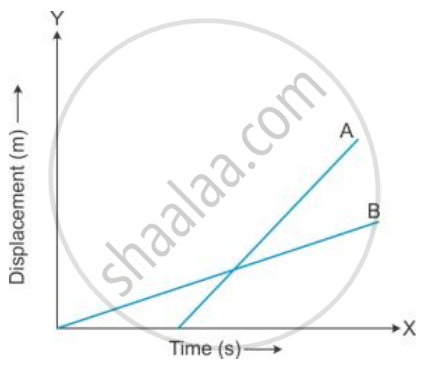Exercise - 2(B) | Q 8 | Page 48

State the type of motion represented by the following sketches in Figures.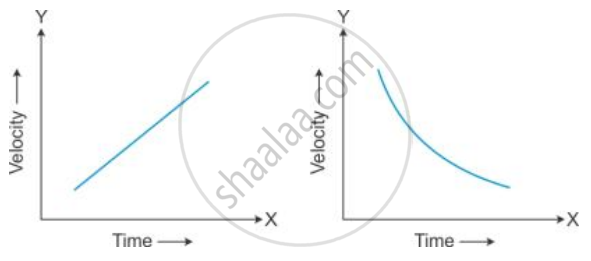Give an example of each type of motion.

Exercise - 2(B) | Q 9 | Page 48

Draw a velocity-time graph for a body moving with an initial velocity u and uniform acceleration a. Use this graph to find the distance travelled by the body in time t.

Exercise - 2(B) | Q 10 | Page 48

What does the slope of velocity-time graph represent?

Exercise - 2(B) | Q 11 | Page 48

Figure shows the velocity-time graphs for two cars A and B moving in the same direction. Which car has greater acceleration? Give reasons to your answer.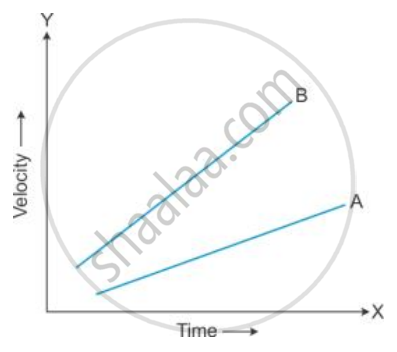Exercise - 2(B) | Q 12 | Page 48

Draw the shape of the velocity-time graph for a body moving with (a) Uniform velocity and (b) Uniform acceleration.

Exercise - 2(B) | Q 13 | Page 48

The velocity-time graph for a uniformly retarded body is a straight line inclined to the time axis with an obtuse angle. How is retardation calculated from the velocity-time graph?

Exercise - 2(B) | Q 14 | Page 48

The figure shows the displacement - time graph for four bodies A, B C and D. In each case state what information do you get about the acceleration (zero, positive or negative).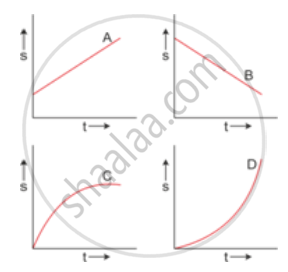Exercise - 2(B) | Q 15 | Page 48

Draw a graph for acceleration against time for a uniformly accelerated motion. How can it be used to find the change in speed in a certain interval of time?

Exercise - 2(B) | Q 16 | Page 48

Draw a velocity-time graph for the free fall of a body under gravity starting from rest. Take g =10m s-2

Exercise - 2(B) | Q 17 | Page 48

How is the distance related with time for motion under uniform acceleration such as the motion of a freely falling body?

Exercise - 2(B) | Q 18 | Page 48

A body falls freely from a certain height. Show graphically the relation between the distance fallen and square of time. How will you determine g from this graph?

### Selina solutions for Concise Physics Class 9 ICSE Chapter 2 Motion in One Dimension Exercise - 2(B) [Page 48]

Exercise - 2(B) | Q 1 | Page 48

The velocity-time graph of a body in motion is a straight line inclined to the time axis. The correct statement is ___________

• Velocity is uniform

• Acceleration is uniform

• Both velocity and acceleration are uniform

• Neither velocity nor acceleration is uniform.

Exercise - 2(B) | Q 2 | Page 48

For a uniformly retarded motion, the velocity-time graph is _____________

• A curve

• A straight line parallel to the time axis

• A straight line perpendicular to the time axis

• A straight line inclined to the time axis

Exercise - 2(B) | Q 3 | Page 48

For uniform motion _________

• The distance-time graph is a straight line parallel to the time axis.

• The speed-time graph is a straight line inclined to the time axis.

• The speed-time graph is a straight line parallel to the time axis.

• The acceleration-time graph is a straight line parallel to the time axis.

### Selina solutions for Concise Physics Class 9 ICSE Chapter 2 Motion in One Dimension Exercise - 2(B) [Pages 49 - 50]

Exercise - 2(B) | Q 1 | Page 49

Figure shows the displacement-time graph for the motion of a body. Use it to calculate the velocity of body at t = 1 s, 2 s and 3 s, and then draw the velocity-time graph in Fig. (b) for it.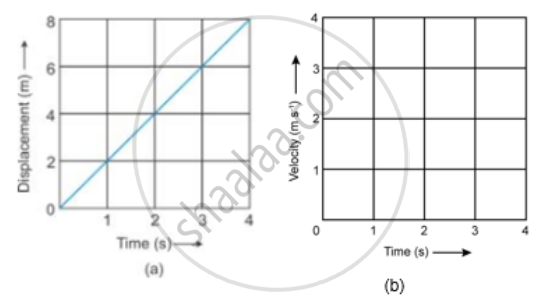Exercise - 2(B) | Q 2 | Page 49

The following table gives the displacement of a car at different instants of time.

 Time (s) 0 1 2 3 4 Displacement (m) 0 5 10 15 20

(a) Draw the displacement-time sketch and find the average velocity of the car.

(b) What will be the displacement of the car at (i) 2.5 s and (ii) 4.5 s?

Exercise - 2(B) | Q 3 | Page 49

A body is moving in a straight line and its displacement at various instants of time is given in the following table

 Time (s) 0 1 2 3 4 5 6 7 Displacement (m) 2 6 12 12 12 18 22 24

Plot the displacement-time graph and calculate

(i) Total distance travelled in the interval 1 s to 5 s.

(ii) Average velocity in time interval 1 s to 5 s.

Exercise - 2(B) | Q 4.1 | Page 49

Figure shows the displacement of a body at different times .

Calculate the velocity of the body as it moves for time interval :
(i) 0 to 5 s,
(ii) 5 s to 7 s
(iii) 7 s to 9 s.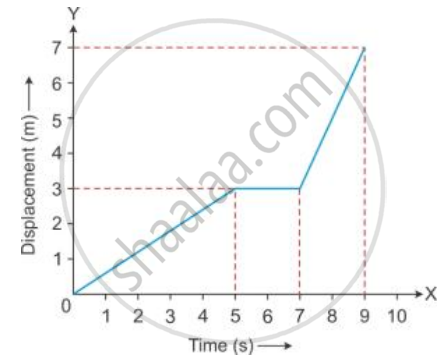Exercise - 2(B) | Q 4.2 | Page 49

Figure shows the displacement of a body at different times .Calculate the average velocity during the time interval 5 s to 9 s ,
[Hint : From 5 s to 9 s , displacement = 7 m - 3m = 4m]

Exercise - 2(B) | Q 5.1 | Page 49

From the displacement-time graph of a cyclist given below in the Figure, find The average velocity in the first 4 s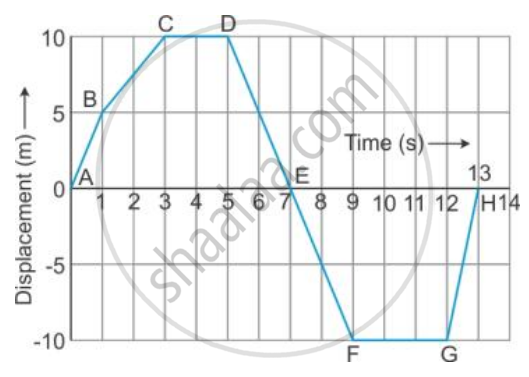Exercise - 2(B) | Q 5.2 | Page 49

From the displacement-time graph of a cyclist given below in the Figure, find The displacement from the initial position at the end of 10 s,Exercise - 2(B) | Q 5.3 | Page 49

From the displacement-time graph of a cyclist given below in the Figure, find The time after which he reaches the starting point .Exercise - 2(B) | Q 6 | Page 49

Figure ahead represents the displacement - time sketch of motion of two cars A and B . Find :
(i) the distance by which the car B was initially ahead of car A.
(ii) the velocities of car A and car B.
(iii) the time in which car A catches car B.
(iv) the distance from start when the car A will catch the car B.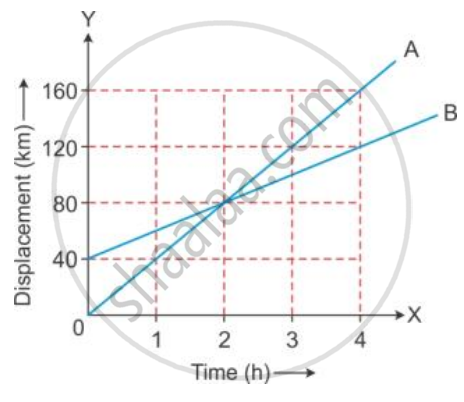Exercise - 2(B) | Q 7 | Page 50

A body at rest is made to fall from the top of a tower . Its displacement at different instants is given in the following table :

 Time (in s) 0.1 0.2 0.3 0.4 0.5 0.6 Displacement (in m) 0.05 0.2 0.45 0.8 1.25 1.8

Draw a displacement - time graph and state whether the motion is uniform or non - uniform ?

Exercise - 2(B) | Q 8 | Page 50

Figure (a) shows the velocity-time graph for the motion of a body. Use it to find the displacement of the body at t = 1 s, 2 s, 3 s and 4 s, then draw on Figure (b), the displacement-time graph for it.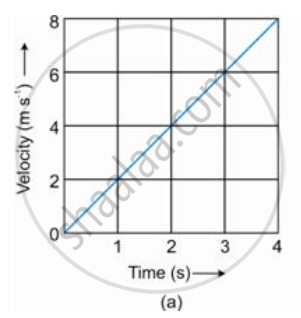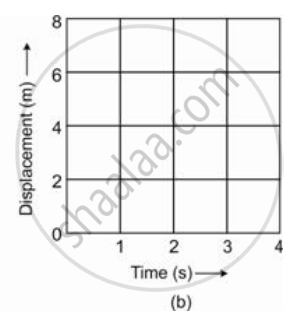Exercise - 2(B) | Q 9.1 | Page 50

Figure given below shows a velocity-time graph for a car starting from rest. The graph has three parts AB, BC and CD.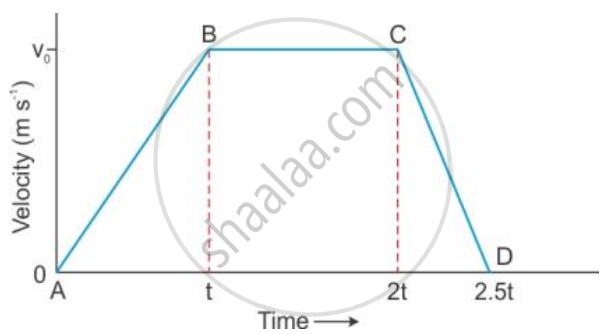State how is the distance travelled in any part determined from this graph .

Exercise - 2(B) | Q 9.2 | Page 50

Figure given below shows a velocity-time graph for a car starting from rest. The graph has three parts AB, BC and CD.Compare the distance travelled in part BC with the distance travelled in part AB.

Exercise - 2(B) | Q 9.3 | Page 50

Figure given below shows a velocity-time graph for a car starting from rest. The graph has three parts AB, BC and CD.Which part of graph shows motion with uniform
(a) velocity (b) acceleration (c) retardation ?

Exercise - 2(B) | Q 9.4 | Page 50

Figure given below shows a velocity-time graph for a car starting from rest. The graph has three parts AB, BC and CD.(a) Is the magnitude of acceleration higher or lower than that of retardation ? Give a reason .
(b) Compare the magnitude of acceleration and retardation .

Exercise - 2(B) | Q 10.1 | Page 50

The velocity-time graph of a moving body is given below in Figure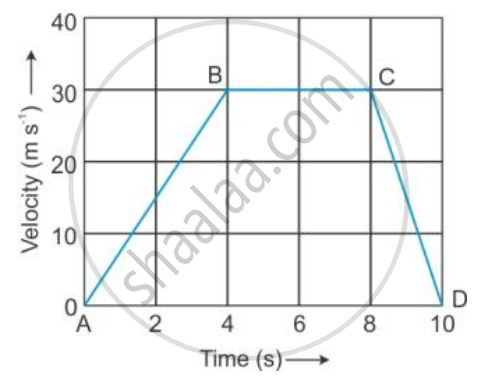The acceleration in parts AB, BC and CD.

Exercise - 2(B) | Q 10.2 | Page 50

The velocity-time graph of a moving body is given below in FigureDisplacement in each part AB, BC and CD.

Exercise - 2(B) | Q 10.3 | Page 50

The velocity-time graph of a moving body is given below in FigureTotal displacement.

Exercise - 2(B) | Q 11 | Page 50

A ball moves on a smooth floor in a straight line with uniform velocity 10 m s-1 for 6 s. At t = 6 s, the ball hits a wall and comes back along the same line to the starting point with the same speed. Draw the velocity-time graph and use it to find the total distance travelled by the ball and its displacement.

Exercise - 2(B) | Q 12 | Page 50

Figure shows the velocity-time graph of a particle moving in a straight line.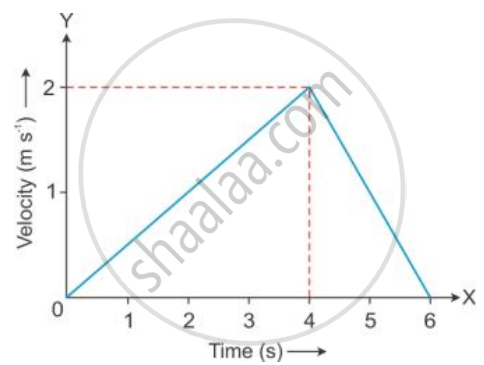(i) State the nature of motion of particle.

(ii) Find the displacement of particle at t = 6 s.

(iii) Does the particle change its direction of motion?

(iv) Compare the distance travelled by the particle from 0 to 4 s and from 4 s to 6 s.

(v) Find the acceleration from 0 to 4 s and retardation from 4 s to 6 s.

### Selina solutions for Concise Physics Class 9 ICSE Chapter 2 Motion in One Dimension Exercise - 2(C) [Page 54]

Exercise - 2(C) | Q 1 | Page 54

Write three equations of uniformly accelerated motion relating the initial velocity (u), final velocity (v), time (t), acceleration (a) and displacement (S).

Exercise - 2(C) | Q 2 | Page 54

Derive the following equations for uniformly accelerated motion:

(i) v = u + at

(ii) "S = ut" + 1/2 "at"^2

(iii) v2 = u2 + 2aS

where the symbols have their usual meanings.

Exercise - 2(C) | Q 3 | Page 54

Write an expression for the distance S covered in time t by a body which is initially at rest and starts moving with a constant acceleration a.

### Selina solutions for Concise Physics Class 9 ICSE Chapter 2 Motion in One Dimension Exercise - 2(C) [Page 54]

Exercise - 2(C) | Q 1 | Page 54

The correct equation of motion is : ___________

• v = u + aS

• v = ut + a

• "S" = ut + 1/2 at

• v = u + at

Exercise - 2(C) | Q 2 | Page 54

A car starting from rest accelerates uniformly to acquire a speed 20 km h-1 in 30 min. The distance travelled by a car in this time interval will be _____________

• 600 km

• 5 km

• 6 km

• 10 km

### Selina solutions for Concise Physics Class 9 ICSE Chapter 2 Motion in One Dimension Exercise - 2(C) [Pages 54 - 55]

Exercise - 2(C) | Q 1 | Page 54

A body starts from rest with uniform acceleration 2 m s-2. Find the distance covered by the body in 2 s.

Exercise - 2(C) | Q 2 | Page 54

A body starts with an initial velocity of 10m s-1 and acceleration 5 m s-2. Find the distance covered by it in 5 s.

Exercise - 2(C) | Q 3 | Page 54

A vehicle is accelerating on a straight road. Its velocity at any instant is 30 km/h. After 2s, it is 33.6 km/h, and after further 2 s, it is 37.2 km/h. Find the acceleration of the vehicle in m s-2. Is the acceleration uniform?

Exercise - 2(C) | Q 4 | Page 54

A body, initially at rest, starts moving with a constant acceleration 2 m s-2. Calculate: (i) the velocity acquired and (ii) the distance travelled in 5 s.

Exercise - 2(C) | Q 5 | Page 54

A bullet initially moving with a velocity 20 m/s strikes a target and comes to rest after penetrating  a  distance  10  cm into the target. Calculate the retardation caused by the target.

Exercise - 2(C) | Q 6 | Page 54

A train moving with a velocity of 20 m s-1 is brought to rest by applying brakes in 5 s. Calculate the retardation.

Exercise - 2(C) | Q 7 | Page 54

A train travels with a speed of 60 km h-1 from station A to station B and then comes back with a speed 80 km h-1 from station B to station A.

Find

(i) The average speed

(ii) The average velocity of the train.

Exercise - 2(C) | Q 8 | Page 54

A train is moving with a velocity of 90 km h-1 . It is brought to stop by applying the brakes which produce a retardation of 0.5 ms-2 Find :
(i) The velocity after 10 s , and
(ii) the time taken by the train to come to rest .

Exercise - 2(C) | Q 9 | Page 54

A car travels a distance 100 m with constant acceleration and average velocity of 20 ms-1. The final velocity acquired by the car is 25 ms-1.

Find

(i) The initial velocity.

(ii) Acceleration of the car.

Exercise - 2(C) | Q 10 | Page 55

When brakes are applied to a bus, retardation produced is 25 cm s-2 and the bus takes 20 s to stop.

Calculate

(i) The initial velocity of the bus

(ii) The distance travelled by the bus during this time.

Exercise - 2(C) | Q 11 | Page 55

A body moves from rest with uniform acceleration and travels 270 m in 3 s. Find the velocity of the body at 10 s after the start.

Exercise - 2(C) | Q 12 | Page 55

A body moving with a constant acceleration travels the distances 3 m and 8 m, respectively in 1 s and 2 s. Calculate:

(i) The initial velocity.

(ii) The acceleration of body.

Exercise - 2(C) | Q 13 | Page 55

A car travels with uniform velocity of 25 m s-1 for 5 s. The brakes are then applied and the car is uniformly retarded and comes to rest in further 10 s. Find: (i) The distance which the car travels before the brakes are applied, (ii) Retardation and (iii) The distance travelled by the car after applying the brakes .

Exercise - 2(C) | Q 14 | Page 55

A space craft flying in a straight course with velocity of 75 km s-1 fires its rocket motors for 6.0 s. At the end of this time its speed is 120 km s-1 in the same direction.

Find

(i) The space craft's average acceleration while the motors were firing

(ii) The distance travelled by the space craft in the first 10 s after the rocket motors were started, the motors being in action for only 6 s.

Exercise - 2(C) | Q 15 | Page 55

A train starts from rest and accelerates uniformly at a rate of 2 m s-2 for 10 s. It then maintains a constant speed for 200 s. The brakes are then applied and the train is uniformly retarded and comes to rest in 50 s. Find

(i) The maximum velocity reached,

(ii) The retardation in the last 50 s,

(iii) The total distance travelled,

(iv) The average velocity of the train.

### Selina solutions for Concise Physics Class 9 ICSE Chapter 2 Motion in One Dimension Extra questions

A body starts to slide over a horizontal surface with an initial velocity of 0.5 ms-1. Due to friction, its velocity decreases at the rate of 0.05 ms-2. How much time will it take for the body to stop?

## Chapter 2: Motion in One Dimension

OthersExercise - 2(A)Exercise - 2(B)Exercise - 2(C)## Selina solutions for Concise Physics Class 9 ICSE chapter 2 - Motion in One Dimension

Selina solutions for Concise Physics Class 9 ICSE chapter 2 (Motion in One Dimension) include all questions with solution and detail explanation. This will clear students doubts about any question and improve application skills while preparing for board exams. The detailed, step-by-step solutions will help you understand the concepts better and clear your confusions, if any. Shaalaa.com has the CISCE Concise Physics Class 9 ICSE solutions in a manner that help students grasp basic concepts better and faster.

Further, we at Shaalaa.com provide such solutions so that students can prepare for written exams. Selina textbook solutions can be a core help for self-study and acts as a perfect self-help guidance for students.

Concepts covered in Concise Physics Class 9 ICSE chapter 2 Motion in One Dimension are Scalar and Vector Quantities, Graphs of Distance-time and Speed-time, Measuring the Rate of Motion - Speed with Direction, Rate of Change of Velocity, Distance and Displacement, Instantaneous Velocity and Speed, Acceleration and Retardation.

Using Selina Class 9 solutions Motion in One Dimension exercise by students are an easy way to prepare for the exams, as they involve solutions arranged chapter-wise also page wise. The questions involved in Selina Solutions are important questions that can be asked in the final exam. Maximum students of CISCE Class 9 prefer Selina Textbook Solutions to score more in exam.

Get the free view of chapter 2 Motion in One Dimension Class 9 extra questions for Concise Physics Class 9 ICSE and can use Shaalaa.com to keep it handy for your exam preparation